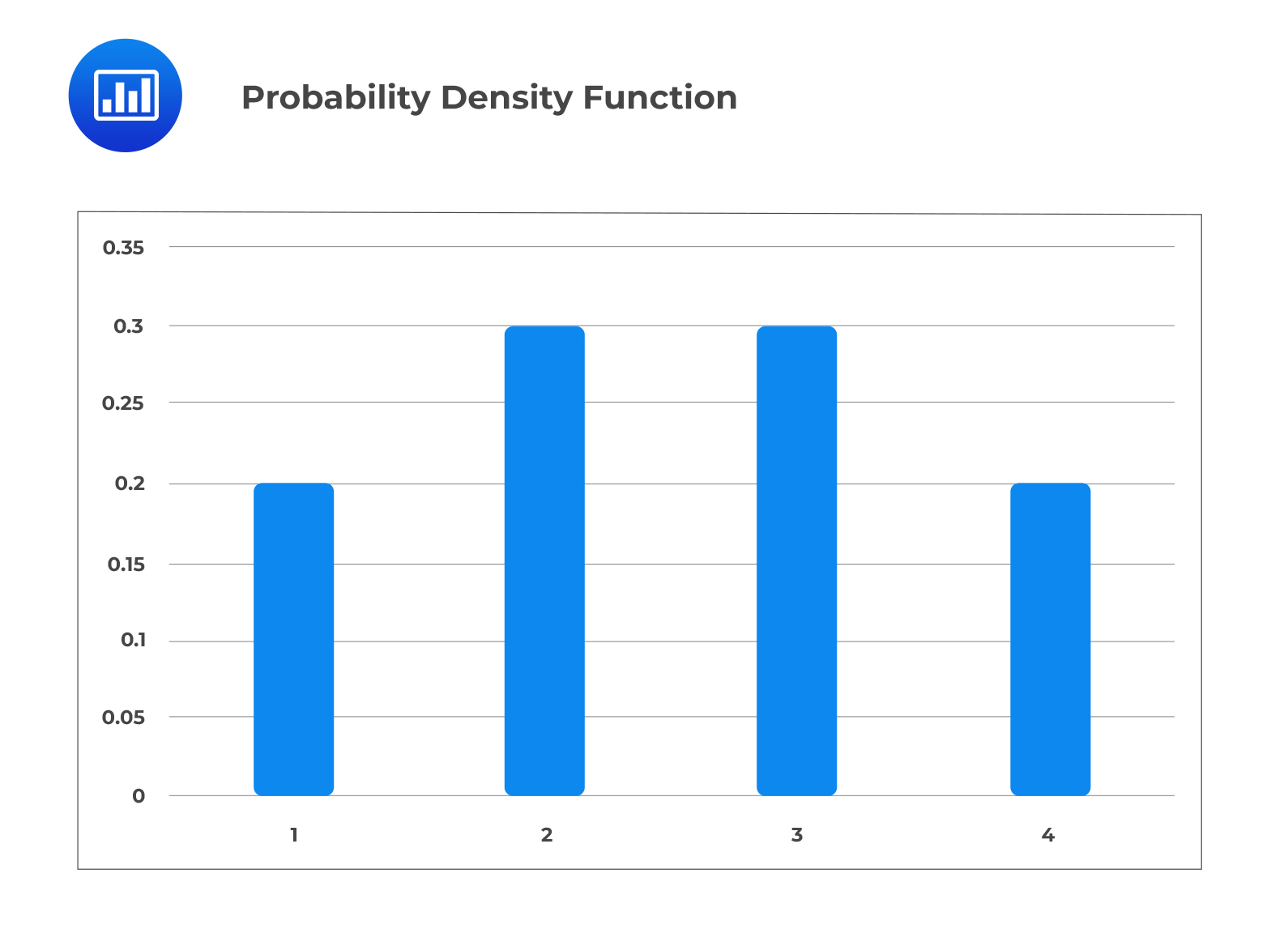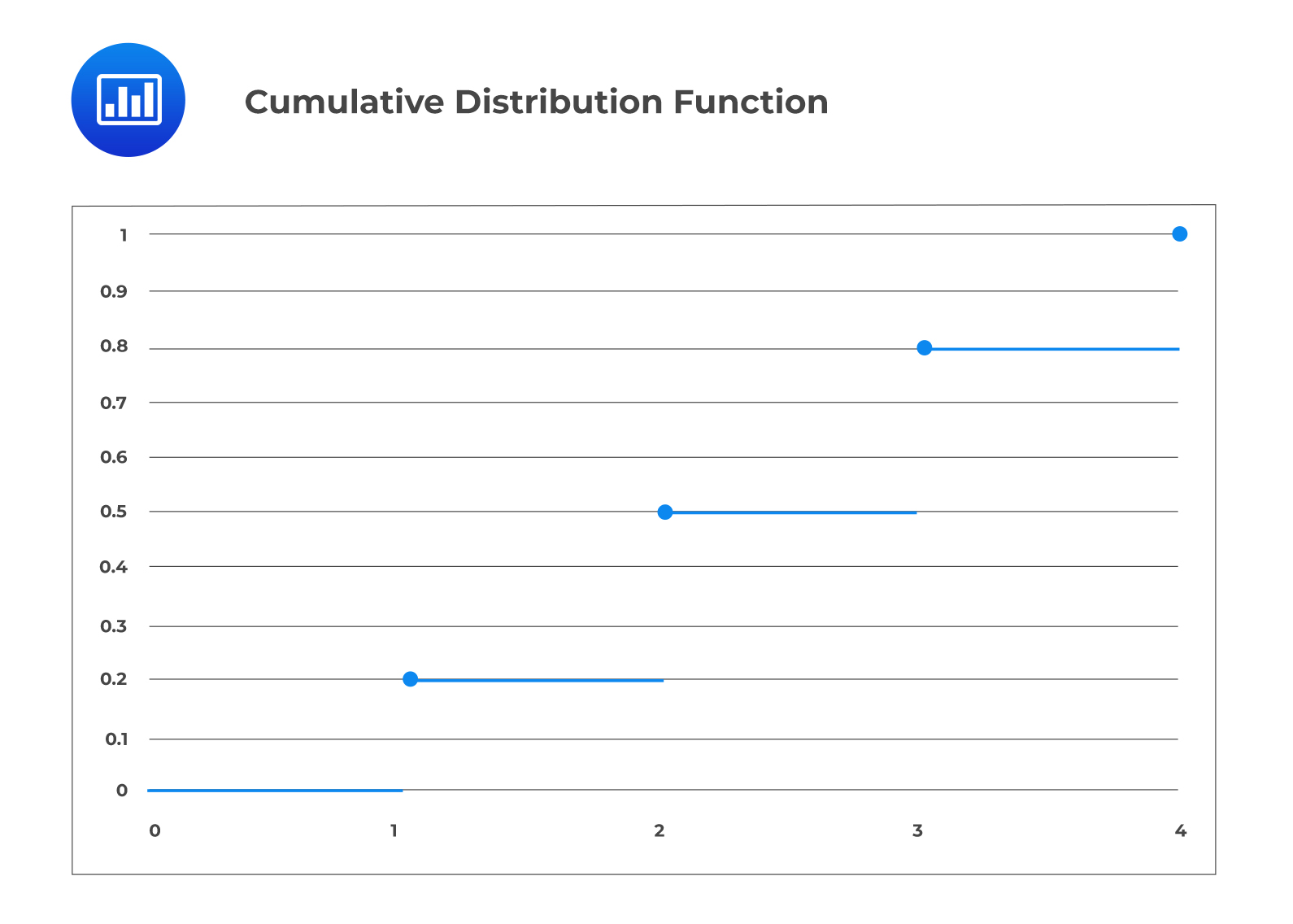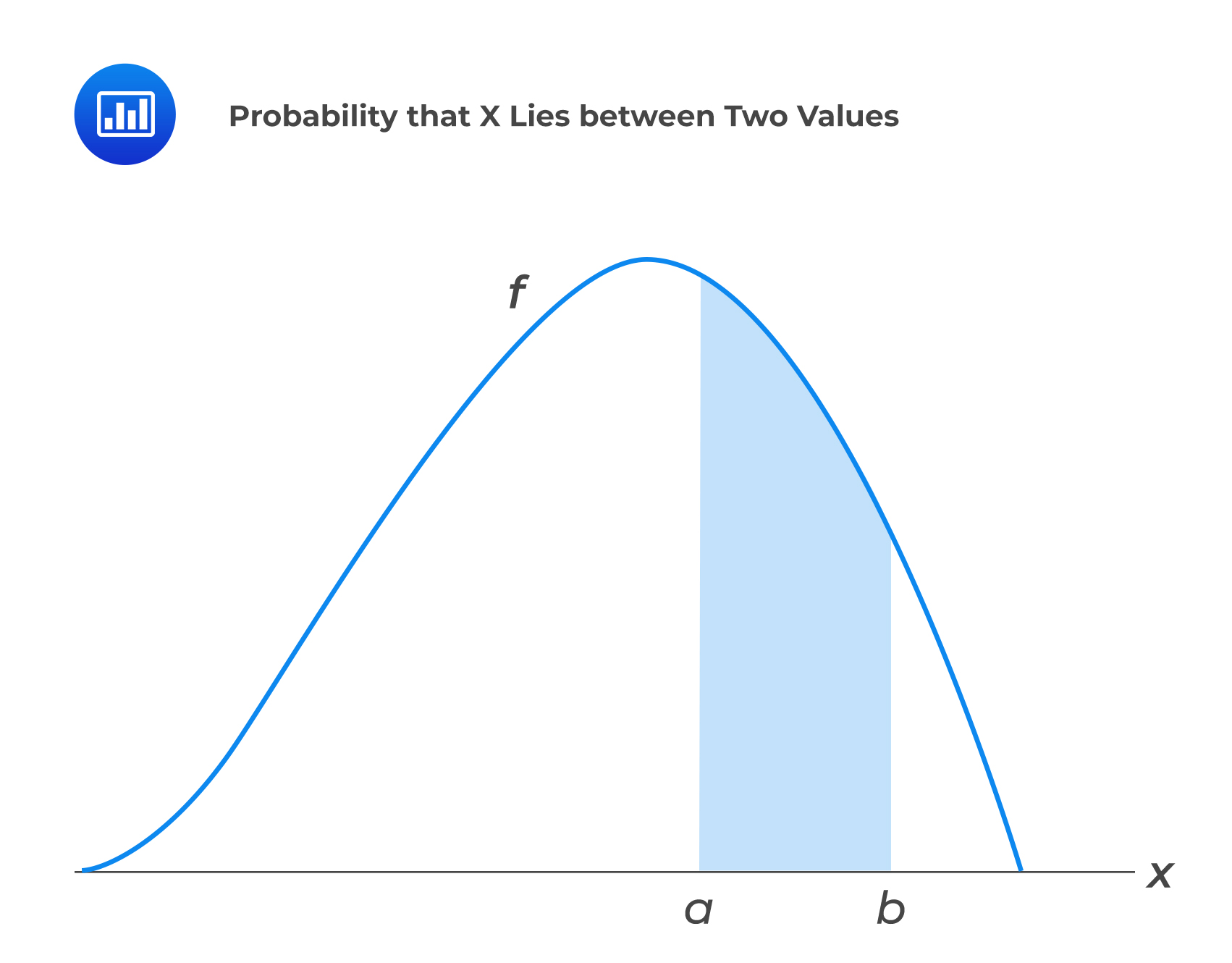Limited Time Offer: Save 10% on all 2022 Premium Study Packages with promo code: BLOG10# Explain and apply the concepts of random variables

Given a random experiment, with sample space, $$S$$, we can define the possible values of $$S$$ as a random variable. Random variables can be discrete or continuous.

## Discrete Random Variables

A discrete random variable is a variable whose range of possible values is from a specified list. In other words,  a discrete take a countable number of positive values in the sample space.

For a discrete variable $$X$$, we define the probability mass function (PMF) as a function that describes the relationship between a random variable, $$X$$, and the probability of each value of $$X$$ occurring. PMF is mathematically defined as:

$$f\left(a\right)=P(X=a)$$

A probability density function (PDF), sometimes called the probability mass function if the variable is discrete, is a function that describes the relationship between a random variable, $$X$$, and the probability of each value of $$x$$ occurring.

The probability mass function is usually presented graphically by plotting $$f(x_i)$$ on the y-axis against $$x_i$$ on the x-axis. For example, the probability massdensity function shown in the graph below can be written as:

$$f\left( x \right) =\begin{cases} 0.2, & x=1,4 \\ 0.3, & x=2,3 \end{cases}$$The probability density (or mass) function of a discrete random variable has the following properties:

1. $$f \left(x \right) > 0,\quad x € S;\quad$$ meaning all individual probabilities must be greater than zero.
2. $${ \Sigma }_{ x\in S }f\left( x \right)=1; \quad$$ meaning the sum of all individual probabilities must equal one.
3. $$P\left( X\in A \right) ={ \Sigma }_{ x\in A }f\left( x \right) \quad$$,where $$A\subset S;\quad$$ meaning the probability of event $$A$$ is the sum of the probabilities of the values of $$X$$ in $$A$$.

## Cumulative Distribution Function for Discrete Random Variables

If we are interested in cumulative probabilities then we can use the cumulative distribution function (CDF) which is defined by the function shown below:

$$F\left( x \right) =P\left( X\le x \right) ,\quad \quad -\infty <x<\infty$$

The cumulative distribution function $$F$$ can be expressed in terms of $$f(a)$$ as:

$$F\left(a\right)=\sum_{\forall\ x\le a}{f(x)}$$

For a discrete random variable X whose possible values are $$x_1,x_2,x_3,\ldots$$ for all $$x_1<x_2<x_3<\ldots$$, the cumulative distribution function $$F$$ of $$X$$ is a step function. As such, $$F$$ is constant in the interval $$[x_{i-1},\ x_i]$$ and take a lip (jump) of $$f(x_i)$$ at $$x_i$$.

#### Example: Graph of Cumulative Distribution Function

Consider the following probability distribution:

$$f(x)=\begin{cases}0.2,&x=1,4\\ 0.3, & x=2,3\\\end{cases}$$

Compute the cumulative distribution and plot its graph.

Solution

Consider the following table:

$$\begin{array}{c|c|c|c} \bf{X_i} & \bf{f(x_i)} & \bf{F(x_i)} \\ \hline 1 & 0.2 & 0.2 \\ \hline 2 & 0.3 & 0.5 \\ \hline 3 & 0.3 & 0.8 \\ \hline 4 & 0.2 & 1.0 \\ \end{array}$$

The cumulative distribution function $$F(x_i)$$ can be written as:

$$F\left( x \right) =\begin{cases} 0, & x<1 \\ 0.2, & 1\le x<2 \\ 0.5, & 2\le x<3 \\ 0.8, & 3\le x<4 \\ 1, & x\ge 4 \end{cases}$$

The graph of the cumulative distribution function is as shown below:

##Continuous Random Variable

A continuous random variable is a random variable that has an infinite number of possible values. For a continuous random variable $$X$$, we define a non-negative function $$f(x)$$ for all $$x\in(-\infty,\infty)$$ with the property that for all real values, $$R$$, so that:

$$\Pr{\left(X\in R\right)}=\int_{R}{f(x)}\ dx$$

The probability density function, $$f(x)$$, of a continuous random variable is a differential equation with the following properties:

1. $$f\left( x \right)\ge 0$$: the function (all probabilities) is always greater than zero.
2. $$\int _{ -\infty }^{ \infty }{ f\left( x \right)dx=1 }$$: the sum of all the possible values (probabilities) is one.

It is important to note that unlike the probability density function of a discrete random variable, the probability density function of a continuous random variable is not a probability. In order to calculate a probability using a probability density function, the following function must be used:

$$P\left( X < a \right) =P\left( X\le a \right) =F\left( a \right) =\int _{ -\infty }^{ a }{ f\left( x \right) dx }$$

Also note that, for $$R=\left[a,b\right]$$

$$P\left( X=a \right) =\int _{ a }^{ a }{ f\left( x \right) dx=0 }$$

The graph  of  $$P\left(a\le X\le b\right)$$ if as shown below:Also, it is important to note that the probability of any individual value of a probability density function is zero, as shown in the formula below:

$$P\left(X=a\right)=\ \int_{a}^{a}{f\left(x\right)}\ dx=0$$

#### Example: Calculating Probability from a PDF

Given the following probability density function of a continuous random variable:

$$f\left( x \right) =\begin{cases} { x }^{ 2 }+c, & 0 < x < 1 \\ 0, & otherwise \end{cases}$$

1. Calculate C.

Solution

\begin{align*}& \int _{ 0 }^{ 1 }{ \left( { x }^{ 2 }+C \right) dx=1 } \\& { \left[ \frac { { x }^{ 3 } }{ 3 } +Cx \right] }_{ x=0 }^{ x=1 }=1 \\& {1}/{3} + C = 1 \\& C = {2}/{3} \\\end{align*}

2. Calculate $$P (X > {\frac {1}{2})}$$.

Solution

\begin{align*}& \int _{ { {1}/{2}} }^{ 1 }{ \left( { x }^{ 2 }+{ {2}/{3}} \right) dx } \\& { \left[ \frac { { x }^{ 3 } }{ 3 } +{ {2}/{3}}x \right] }_{ x={\frac {1}{2}} }^{ x=1 } \\& \left[ {1}/{3}+{2}/{3} \right] – \left[{1}/{24}+{1}/{3} \right] = {5}/{8} \\\end{align*}

## Conditional Probability

Conditional probabilities of random variables can be calculated in a similar way to empirical conditional probabilities, as shown in the formula below:

$$P\left( A|B \right) =\frac { P\left( A\cap B \right) }{ P\left( B \right) }$$

Let event $$A$$ be $$X > 2$$ and event $$B$$ be $$X < 4$$ then

$$P\left( A|B \right) =\frac { P\left( 2<X<4 \right) }{ P\left( X<4 \right) }$$

#### Example: Conditional Probability

Given the following probability density function of a continuous random variable:

$$f\left( x \right) =\begin{cases} { x }^{ 2 }+{2}/{3}, & 0 < x < 1 \\ 0, & otherwise \end{cases}$$

Calculate $${P} \left({ {X} }>\cfrac{3}{4}∣{ {X} }>\cfrac{1}{2}\right)$$

#### Solution

Using the fact that:

$$P\left(A\mid B\right)=\frac{P\left(A\cap B\right)}{P(B)}$$

We have,

\begin{align} P(X>\frac{3}{4}\mid X>\frac{1}{2})\ &=\ \frac{P(X>\frac{3}{4}\cap X>\frac{1}{2})\ }{P(X>\frac{1}{2})}\\ &=\frac{P\left(X>\frac{3}{4}\right)}{P(X > \frac{1}{2})}\end{align}

Now.

\begin{align*} P\left(X>\frac{3}{4}\right) & =\int _{ { \frac{3}{4} } }^{ 1}{\left({ {x} }^{2}+\cfrac{2}{3}\right) {dx} } \\ & = 0.359375 \\ {P}\left({ {X} }>\cfrac{1}{2}\right) & =\int _{ { \frac{1}{2} } }^{ 1 }{ \left({ {x} }^{2}+\cfrac{2}{3}\right) {dx} }=\left[ \cfrac{ { {x} }^{3} }{3}+\cfrac{2}{3} { {x} } \right]_{{ {x} }=\frac{1}{2}}^{{ {x} } = 1} =\cfrac{5}{8} \\ \Rightarrow {P} \left( { {X} } > \cfrac{3}{4}|{ {X} }>\cfrac{1}{2}\right) &= \cfrac{0.359375}{\cfrac{5}{8}} =0.575 \end{align*}

Learning Outcome

Topic 2.a – b: Univariate Random Variables – Explain and apply the concepts of random variables, probability and probability density functions, cumulative distribution functions & Calculate conditional probabilities.

Shop CFA® Exam Prep

Offered by AnalystPrepLevel I
Level II
Level III
All Three Levels
Featured Shop FRM® Exam PrepFRM Part I
FRM Part II
FRM Part I & Part II
Learn with Us

Subscribe to our newsletter and keep up with the latest and greatest tips for success
Shop Actuarial Exams PrepExam P (Probability)
Exam FM (Financial Mathematics)
Exams P & FM
Shop GMAT® Exam PrepComplete CourseDaniel Glyn
2021-03-24
I have finished my FRM1 thanks to AnalystPrep. And now using AnalystPrep for my FRM2 preparation. Professor Forjan is brilliant. He gives such good explanations and analogies. And more than anything makes learning fun. A big thank you to Analystprep and Professor Forjan. 5 stars all the way!michael walshe
2021-03-18
Professor James' videos are excellent for understanding the underlying theories behind financial engineering / financial analysis. The AnalystPrep videos were better than any of the others that I searched through on YouTube for providing a clear explanation of some concepts, such as Portfolio theory, CAPM, and Arbitrage Pricing theory. Watching these cleared up many of the unclarities I had in my head. Highly recommended.Nyka Smith
2021-02-18
Every concept is very well explained by Nilay Arun. kudos to you man!2021-02-13